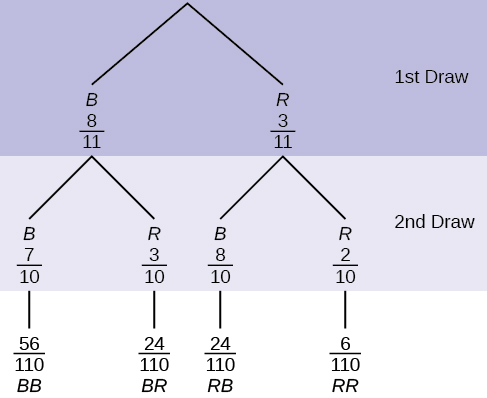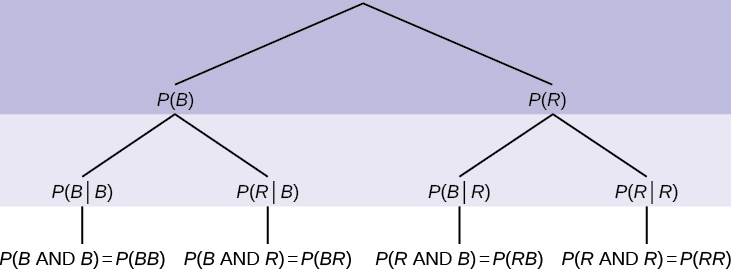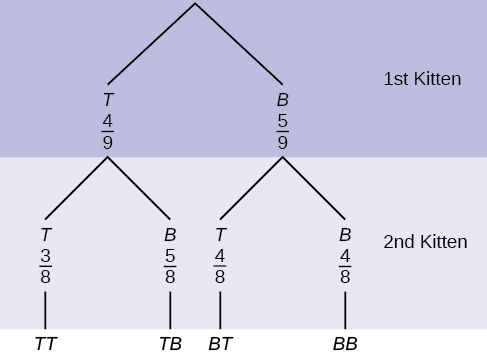## 3.5 Tree and Venn Diagrams

Sometimes, when the probability problems are complex, it can be helpful to graph the situation. Tree diagrams and Venn diagrams are two tools that can be used to visualize and solve conditional probabilities.

## Tree Diagrams

A tree diagram is a special type of graph used to determine the outcomes of an experiment. It consists of “branches” that are labeled with either frequencies or probabilities. Tree diagrams can make some probability problems easier to visualize and solve. The following example illustrates how to use a tree diagram.

## Example 1

In an urn, there are 11 balls. Three balls are red (R) and eight balls are blue (B). Draw two balls, one at a time, with replacement. “With replacement” means that you put the first ball back in the urn before you select the second ball.

a. Use tree diagram to show all possible outcomes.

b. List the 24 BR outcomes.

c. Using the tree diagram, calculate P(RR).

d. Using the tree diagram, calculate P(RB OR BR).

e. Using the tree diagram, calculate P(R on 1st draw AND B on 2nd draw).

f. Using the tree diagram, calculate P(R on 2nd draw GIVEN B on 1st draw).

g. Using the tree diagram, calculate P(BB).

h. Using the tree diagram, calculate P(B on the 2nd draw given R on the first draw).

### Try It

In a standard deck, there are 52 cards. 12 cards are face cards (event F) and 40 cards are not face cards (event N). Draw two cards, one at a time, with replacement. All possible outcomes are shown in the tree diagram as frequencies. Using the tree diagram, calculate P(FF).

“Without replacement” means that you do not put the first ball back before you select the second marble. Following is a tree diagram for this situation. The branches are labeled with probabilities instead of frequencies. The numbers at the ends of the branches are calculated by multiplying the numbers on the two corresponding branches.

## Example 2

An urn has three red marbles and eight blue marbles in it. Draw two marbles, one at a time, this time without replacement, from the urn.Total = $\displaystyle\frac{{56+24+24+6}}{{110}}=\frac{{110}}{{110}}=1$
 Note:If you draw a red on the first draw from the three red possibilities, there are two red marbles left to draw on the second draw. You do not put back or replace the first marble after you have drawn it. You draw without replacement, so that on the second draw there are ten marbles left in the urn.

a. P(RR) = ________

b. Fill in the blanks:

P(RB or BR) = ____________

c. P(R on 2nd|B on 1st) = ___________

d. Fill in the blanks.

P(R on 1st AND B on 2nd) = P(RB) = (___)(___) = $\frac{24}{100}$

e. Find P(BB).

f. Find P(B on 2nd|R on 1st).

If we are using probabilities, we can label the tree in the following general way.• P(R|R) here means P(R on 2nd|R on 1st)
• P(B|R) here means P(B on 2nd|R on 1st)
• P(R|B) here means P(R on 2nd|B on 1st)
• P(B|B) here means P(B on 2nd|B on 1st)

### Try It

A litter of kittens available for adoption at the Humane Society has four tabby kittens and five black kittens. A family comes in and randomly selects two kittens (without replacement) for adoption.1. What is the probability that both kittens are tabby?

2. What is the probability that one kitten of each coloring is selected?

3. What is the probability that a tabby is chosen as the second kitten when a black kitten was chosen as the first?

4. What is the probability of choosing two kittens of the same color?

## Example 3

Suppose there are 4 red balls and 3 yellow balls in a box. Two balls are drawn from the box without replacement. What is the probability that one ball of each coloring is selected?

## Venn Diagram

A Venn diagram is a picture that represents the outcomes of an experiment. It generally consists of a box that represents the sample space S together with circles or ovals. The circles or ovals represent events.

## Example 4

Suppose an experiment has the outcomes 1, 2, 3, … , 12 where each outcome has an equal chance of occurring.
Let event A = {1, 2, 3, 4, 5, 6} and event B = {6, 7, 8, 9}. Then A AND B = {6} and A OR B = {1, 2, 3, 4, 5, 6, 7, 8, 9}.
The Venn diagram is as follows:

### Try It

Suppose an experiment has outcomes black, white, red, orange, yellow, green, blue, and purple, where each outcome has an equal chance of occurring. Let event C = {green, blue, purple} and event P = {red, yellow, blue}. Then C AND P = {blue} and C OR P = {green, blue, purple, red, yellow}. Draw a Venn diagram representing this situation.

## Example 5

Flip two fair coins. Let A = tails on the first coin. Let B = tails on the second coin. Then A = {TT, TH} and B = {TT, HT}. Therefore,A AND B = {TT}. A OR B = {TH, TT, HT}.

The sample space when you flip two fair coins is X = {HH, HT, TH, TT}. The outcome HH is in NEITHER A NOR B. The Venn diagram is as follows:

### Try It

Roll a fair, six-sided die. Let A = a prime number of dots is rolled. Let B = an odd number of dots is rolled. Then A = {2, 3, 5} and B = {1, 3, 5}. Therefore, A AND B = {3, 5}. A OR B = {1, 2, 3, 5}. The sample space for rolling a fair die is S = {1, 2, 3, 4, 5, 6}. Draw a Venn diagram representing this situation.

## Example 6

40% of the students at a local college belong to a club and 50% work part time. 5% of the students work part time and belong to a club.

a. Draw a Venn diagram showing the relationships.

b. If a student is selected at random, find the probability that the student belongs to a club.

c. If a student is selected at random, find the probability that the student works part time.

d. If a student is selected at random, find the probability that the student belongs to a club AND works part time.

e. If a student is selected at random, find the probability that the student belongs to a club given that the student works part time.

f. If a student is selected at random, find the probability that the student belongs to a club OR works part time.

### Try It

Fifty percent of the workers at a factory work a second job, 25% have a spouse who also works, 5% work a second job and have a spouse who also works. Draw a Venn diagram showing the relationships. Let W = works a second job and S = spouse also works.

Example 7

In a bookstore, the probability that the customer buys a novel is 0.6, and the probability that the customer buys a non-fiction book is 0.4. Suppose that the probability that the customer buys both is 0.2.

1. Draw a Venn diagram representing the situation.

2. Find the probability that the customer buys either a novel or anon-fiction book.

3. In the Venn diagram, describe the overlapping area using a complete sentence.

4. Suppose that some customers buy only compact disks. Draw an oval in your Venn diagram representing this event.

https://youtu.be/MassxXy8iko

## Glossary

Tree Diagram
the useful visual representation of a sample space and events in the form of a “tree” with branches marked by possible outcomes together with associated probabilities (frequencies, relative frequencies)
Venn Diagram
the visual representation of a sample space and events in the form of circles or ovals showing their intersections
Solutions to Try These 1:
a. B1R1 B1R2 B1R3 B2R1 B2R2 B2R3 B3R1 B3R2 B3R3 B4R1 B4R2 B4R3 B5R1 B5R2 B5R3 B6R1 B6R2 B6R3 B7R1B7R2 B7R3 B8R1 B8R2 B8R3
b. P(RR) = (311)(311) = 9121
c. P(RB OR BR) = (311)(811) + (811)(311) = 48121
d. P(R on 1st draw AND B on 2nd draw) = P(RB) = (311)(811) = 24121

e. P(R on 2nd draw GIVEN B on 1st draw) = P(R on 2nd|B on 1st) = 2488 = 311

This problem is a conditional one. The sample space has been reduced to those outcomes that already have a blue on the first draw. There are 24 + 64 = 88 possible outcomes (24 BR and 64 BB). Twenty-four of the 88 possible outcomes are BR. 2488 = 311.

f. P(BB) = 64121

g. P(B on 2nd draw|R on 1st draw) = 811

There are 9 + 24 outcomes that have R on the first draw (9 RR and 24 RB). The sample space is then 9 + 24 = 33. 24 of the 33 outcomes have B on the second draw. The probability is then 2433.

Solutions to Try These 2:

a. P(RR) = (311)(210)=6110
b. P(RB OR BR) = (311)(810) + (811)(310) = 48110
c. P(R on 2nd|B on 1st) = 310
d. P(R on 1st AND B on 2nd) = P(RB) = (311)(810) = 24100
e. P(BB) = (811)(710)
f. Using the tree diagram, P(B on 2nd | R on 1st) = P(B) = 810.